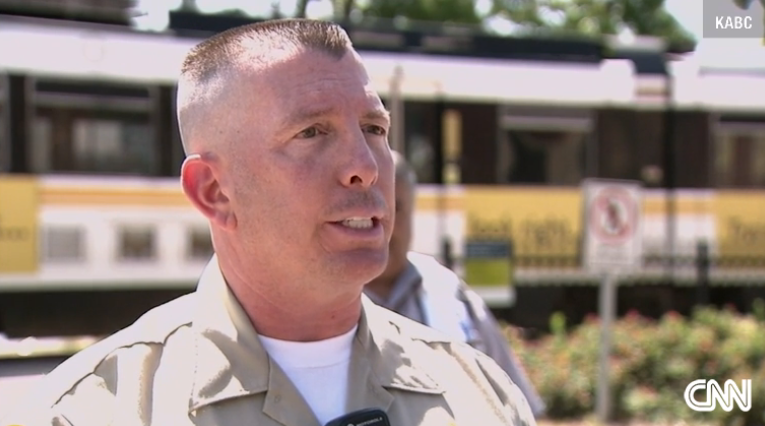## Saturday, March 28, 2015

### 74 93 113 | The Los Angeles Train-Car Accident of March 28, 2015

This story comes on the account of Michael Verlich.
• Michael = 4+9+3+8+1+5+3 = 33
• Verlich = 4+5+9+3+9+3+8 = 41
• Michael Verlich = 74
The site of the collision is interesting.
• Watt = 23+1+20+20 = 64
• Way = 23+1+25 = 49
• Watt Way = 113
• Exposition = 5+24+16+15+19+9+20+9+15+14 = 146
• Boulevard = 2+15+21+12+5+22+1+18+4 = 100
• Exposition Boulevard = 246
• Watt = 5+1+2+2 = 10
• Way = 5+1+7 = 13
• Watt Way = 23
• Exposition = 5+6+7+6+1+9+2+9+6+5 = 56
• Boulevard = 2+6+3+3+5+4+1+9+4 37
• Exposition Boulevard = 93
Where have we seen these numbers before?  Never mind the 21 injured...
• L = 12
• A = 1
• LA = 121
• Revelation = 121 (Simple English Gematria)
• Twenty One = 2+5+5+5+2+7+6+5+5 = 42
One last point, the Hyundai mentioned in the story also has familiar Gematria.
• Hyundai = 8+25+21+14+4+1+9 = 82
• Hyundai = 8+7+3+5+4+1+9 = 37Michael Verlich, AKA Lying Asshole